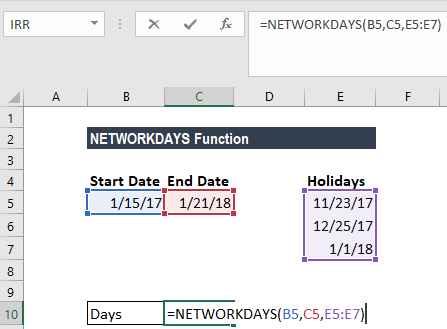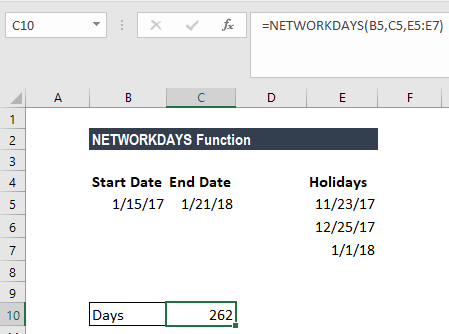# NETWORKDAYS Function

Get the number of workdays between two given dates

## How to calculate working days in Excel

The NETWORKDAYS Function calculates the number of workdays between two dates in Excel. When using the function, the number of weekends are automatically excluded. It also allows you to skip specified holidays and only count business days. It is categorized in Excel as a Date/Time Function. You can use this formula to calculate working days in Excel between two dates.

In finance and accounting, the net workdays function is useful in calculating employee benefits that accrue based on days worked, the number of working days available during a project, or the number of business days required to resolve a ticket raised by a customer, etc.

### Formula

=NETWORKDAYS(start_date, end_date, [holidays])

The function uses the following arguments:

1. Start_date (required argument) – Start_date can either be earlier than end_date, later than end_date, or same as end_date.
2. End_date (required argument) – The end date.
3. Holidays (optional argument) – This specifies the list of holidays that should be excluded from the work days calculation. We can enter it as a range of cells that contain the holiday dates (that is F2:F4) or as a list of serial numbers that represent the holiday dates.

It is recommended that the start_date, end_date and [holidays] arguments are entered as either:

• References to cells containing dates; or
• Dates returned from formulas.

### How to calculate NETWORKDAYS Function in Excel?

Let’s see a few examples to understand how the NETWORKDAYS function to calculate working days in Excel.

#### Example 1 – Working days between two dates

Suppose we wish to calculate the workdays or business days from January 15, 2017 to January 21, 2018. The holidays in between are:

1. November 23, 2017 – Thanksgiving Day
2. December 25, 2017 – Christmas Day
3. January 1, 2018 – New Year’s Day

To calculate the number of working days, we use the following function:Using the formula above, we will get the number of working days as 262, as shown below.### Pointers about the NETWORKDAYS function

1. Negative return value – If the start date is later than the end date, the function will return a negative value.
2. The NETWORKDAYS function will include both the start date and end date when calculating work days. So, if we give NETWORKDAYS the same date for start date and end date, it will return 1.
3. #VALUE! error – Occurs when any of the given arguments is not a valid date.
4. If we wish to use weekends other than Saturday and Sunday, we need to use the NETWORKDAYS.INTL function.

Thanks for reading CFI’s guide to important Excel functions! By taking the time to learn and master these functions, you’ll significantly speed up your financial analysis. To learn more, check out these additional CFI resources:

• Excel Functions for Finance